# RS Aggarwal Solutions for Class 7 Maths Chapter 8 Ratio and Proportion

RS Aggarwal Solutions for Class 7 Maths Chapter 8 – Ratio and Proportion is the best study material for those students who are finding difficulties in solving problems. These solutions can help students to clear doubts quickly and help in understanding the topic effectively. Our expert tutors formulate these exercises to assist you with your exam preparation to attain good marks in Maths. Students who wish to score good marks in Maths practise RS Aggarwal Class 7 Solutions.

These Ratio and Proportion solutions are available for download in pdf format and provide solutions for all the questions provided in Class 7 Maths Chapter 8 wherein problems are solved step by step with detailed explanations. Download pdf of Class 7 Chapter 8 in their respective links.

## Download PDF of RS Aggarwal Solutions for Class 7 Maths Chapter 8 – Ratio and Proportion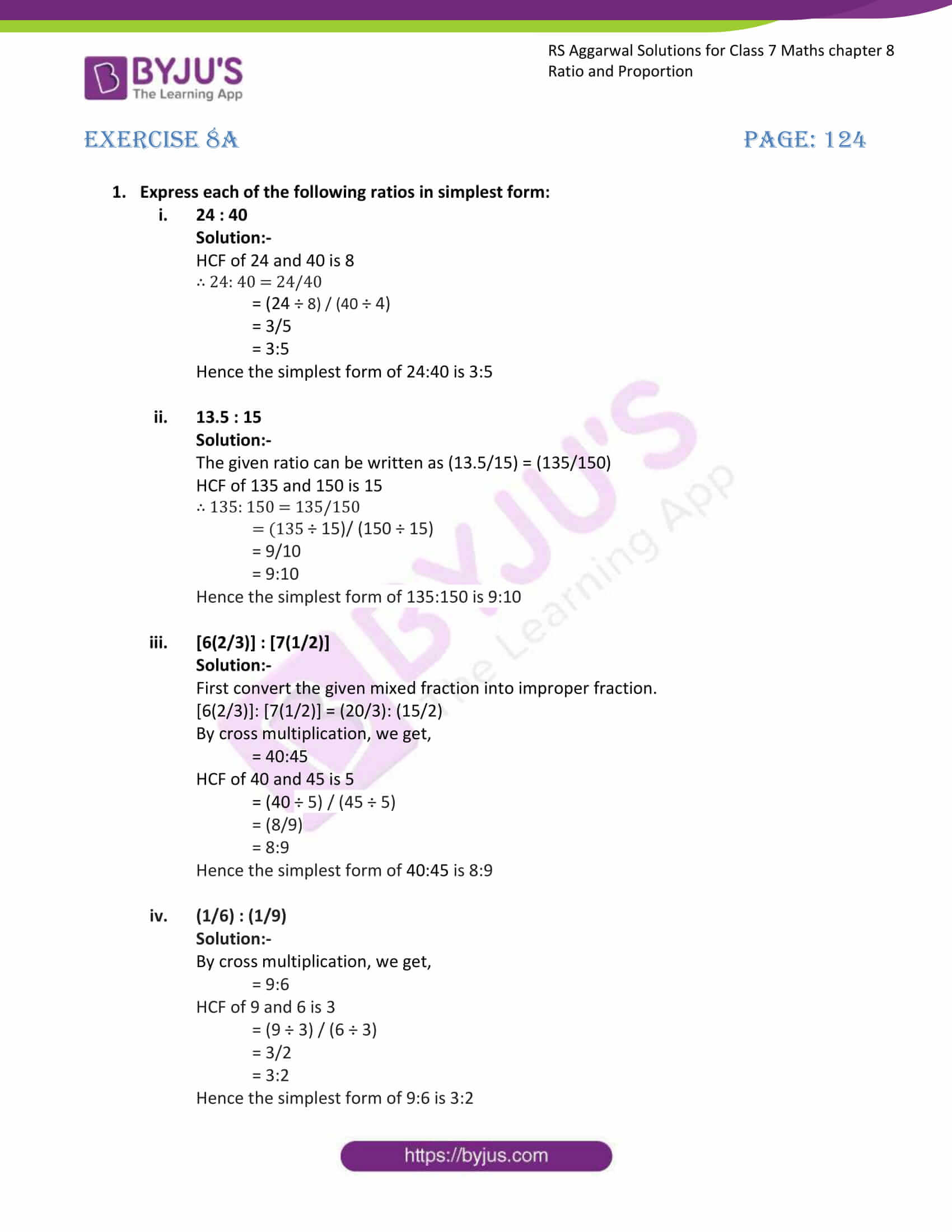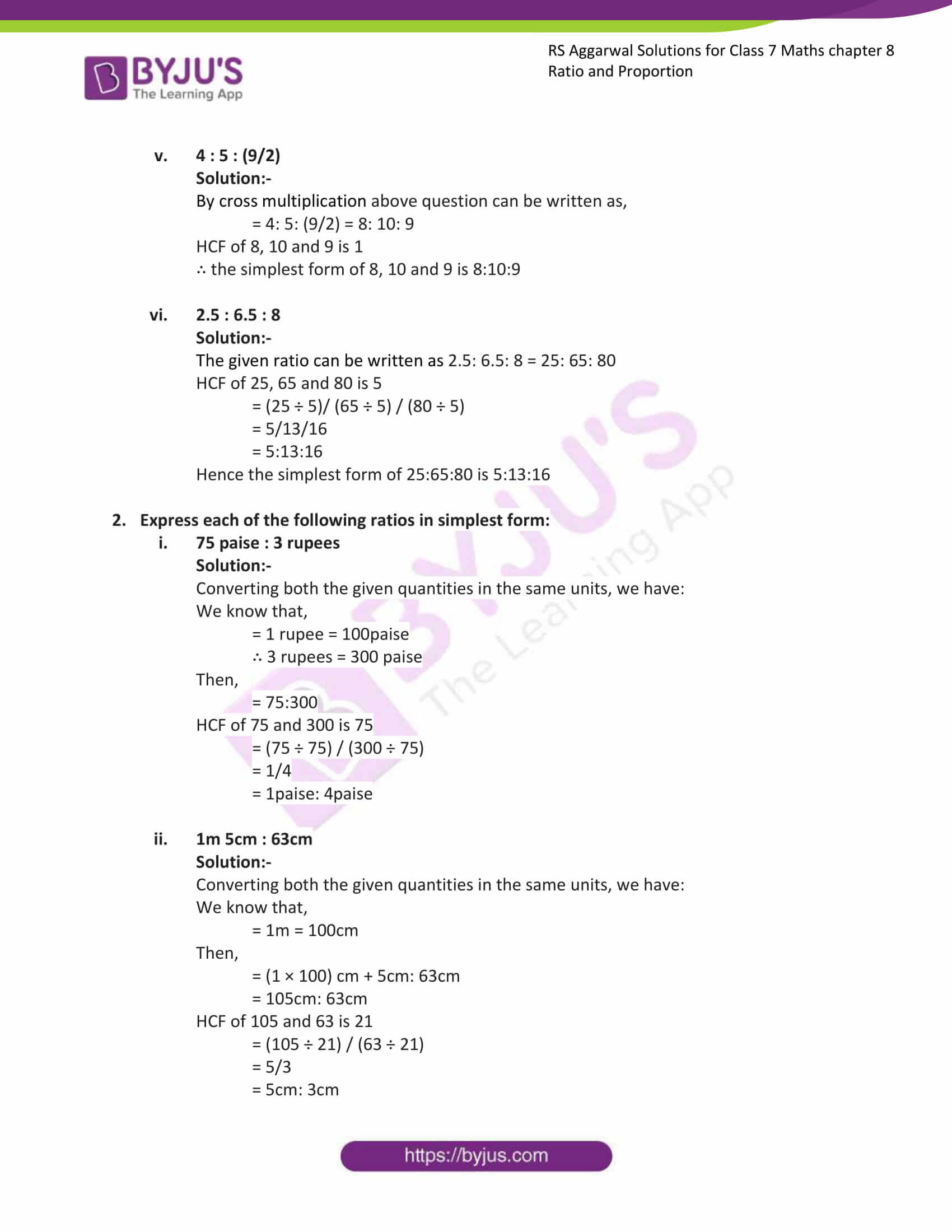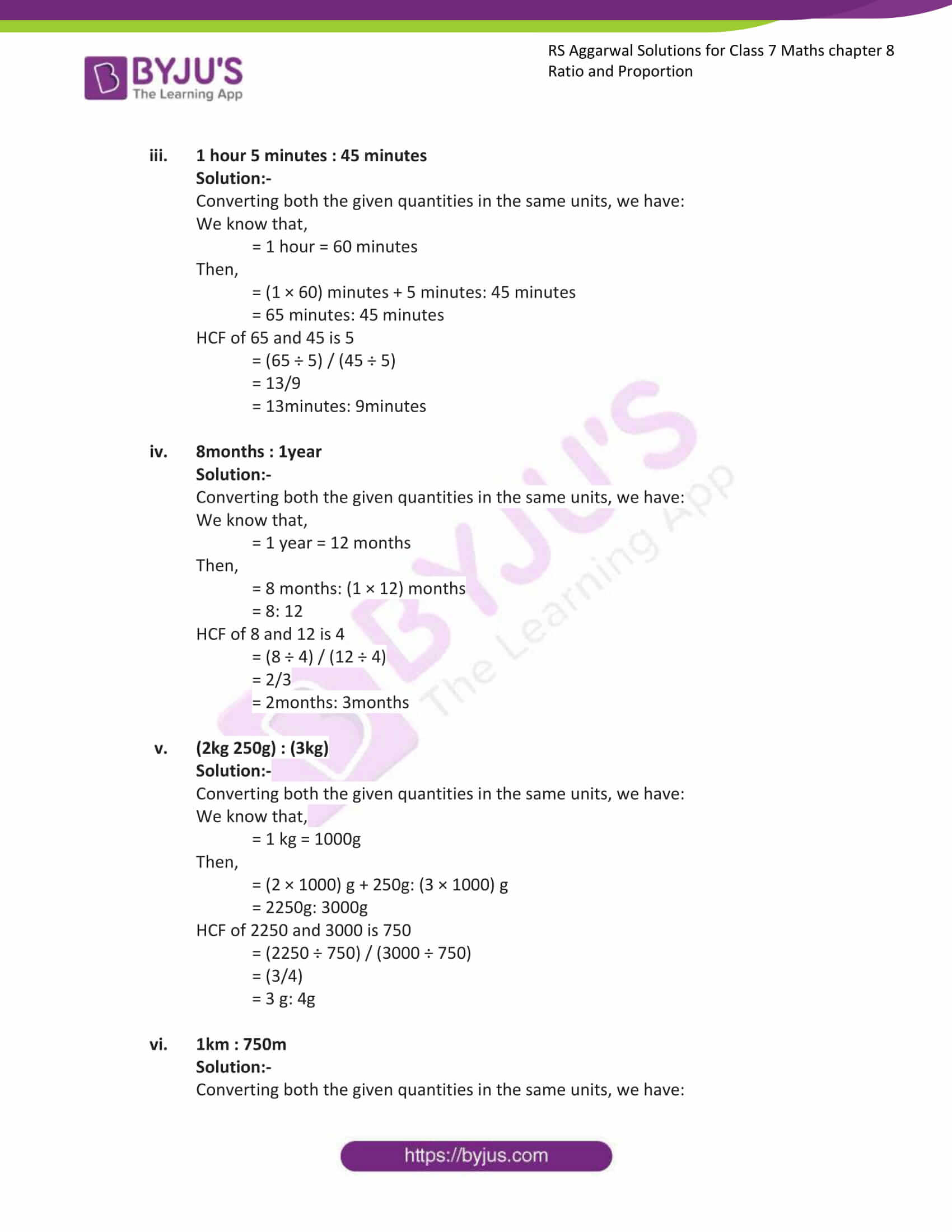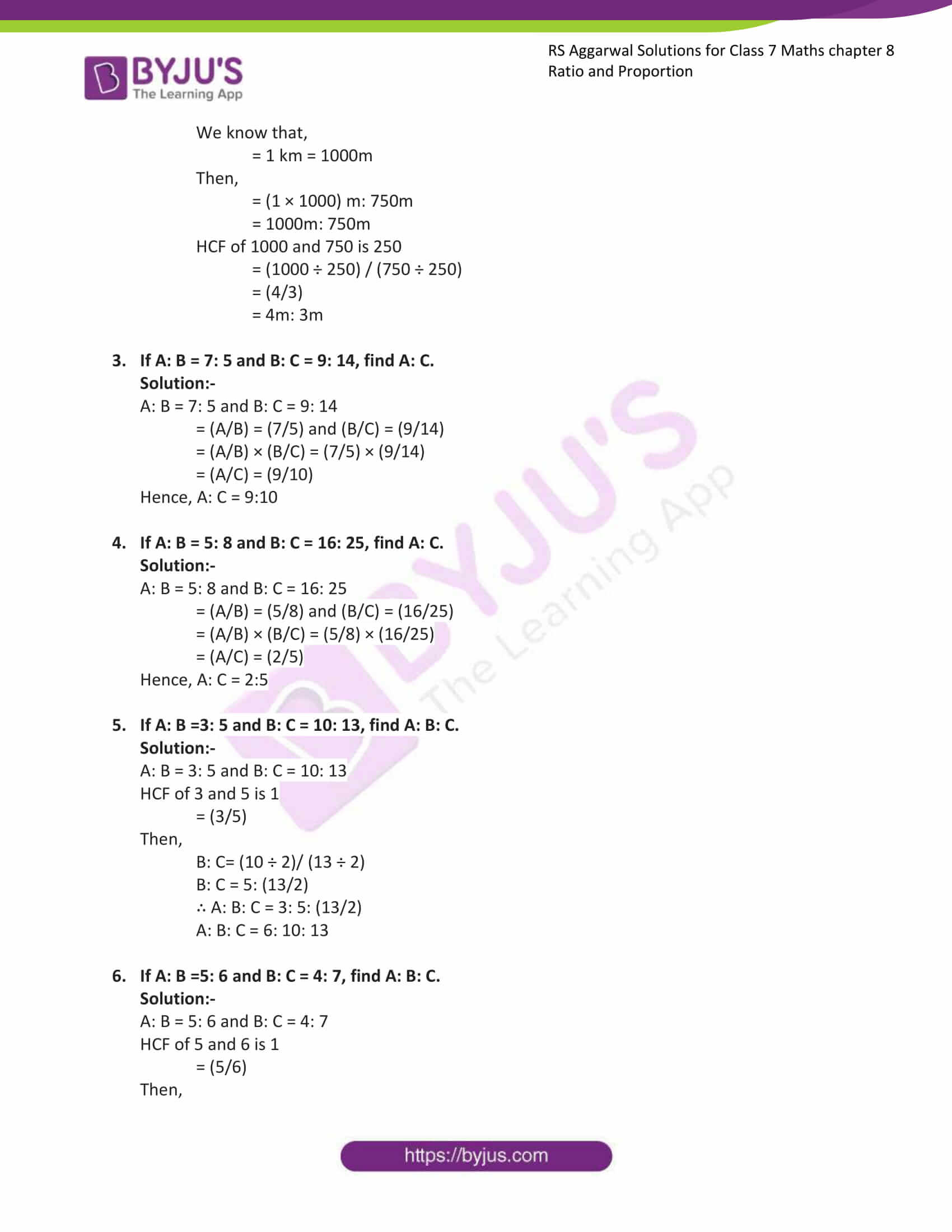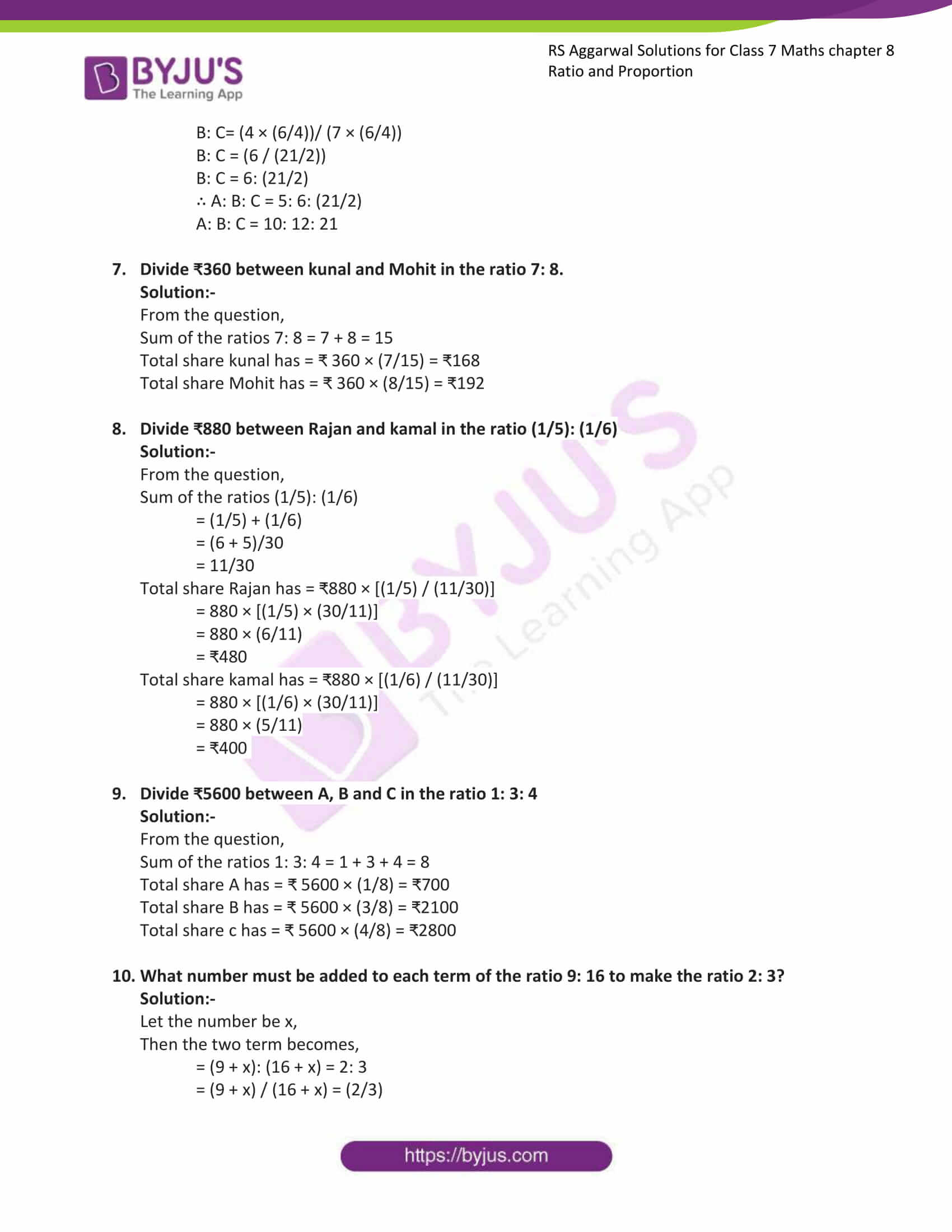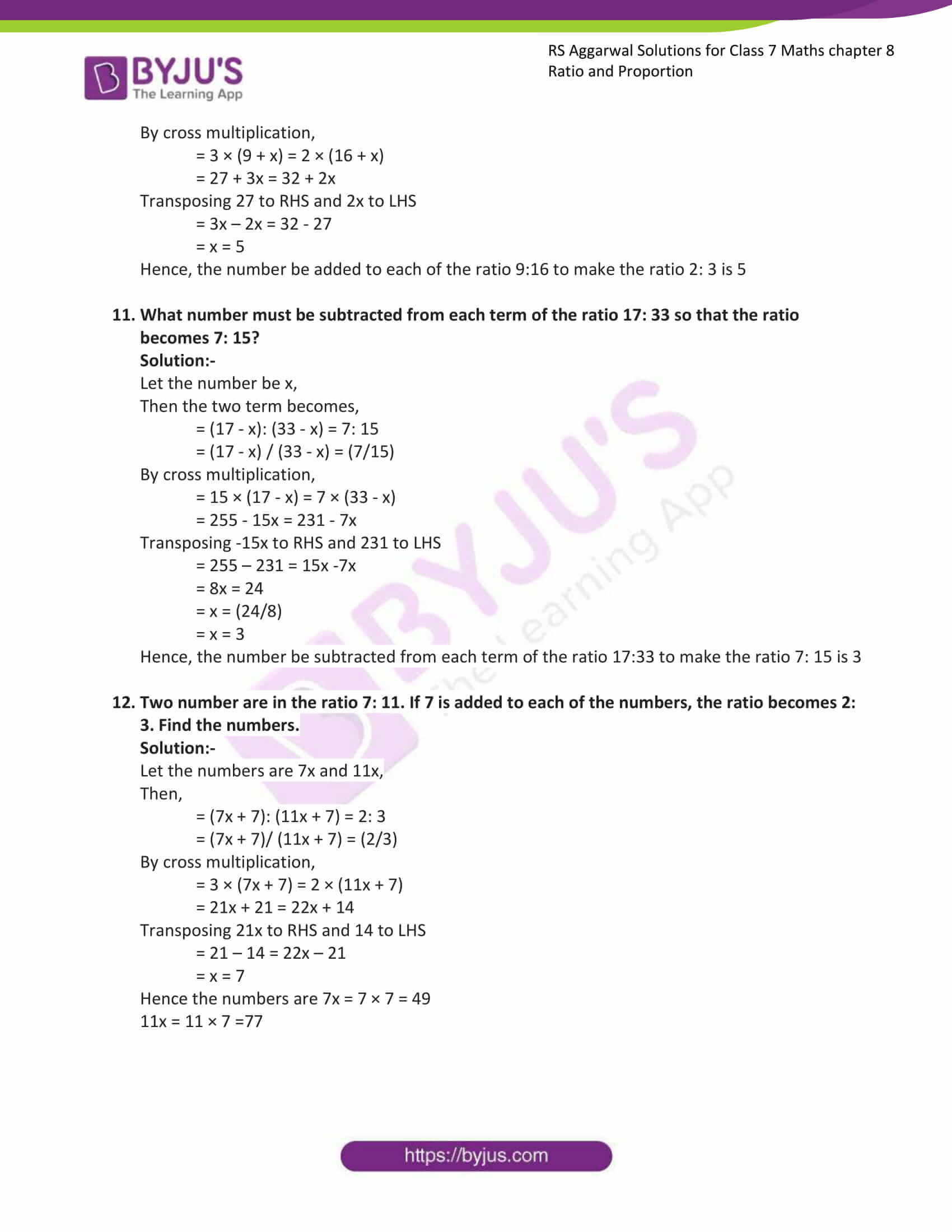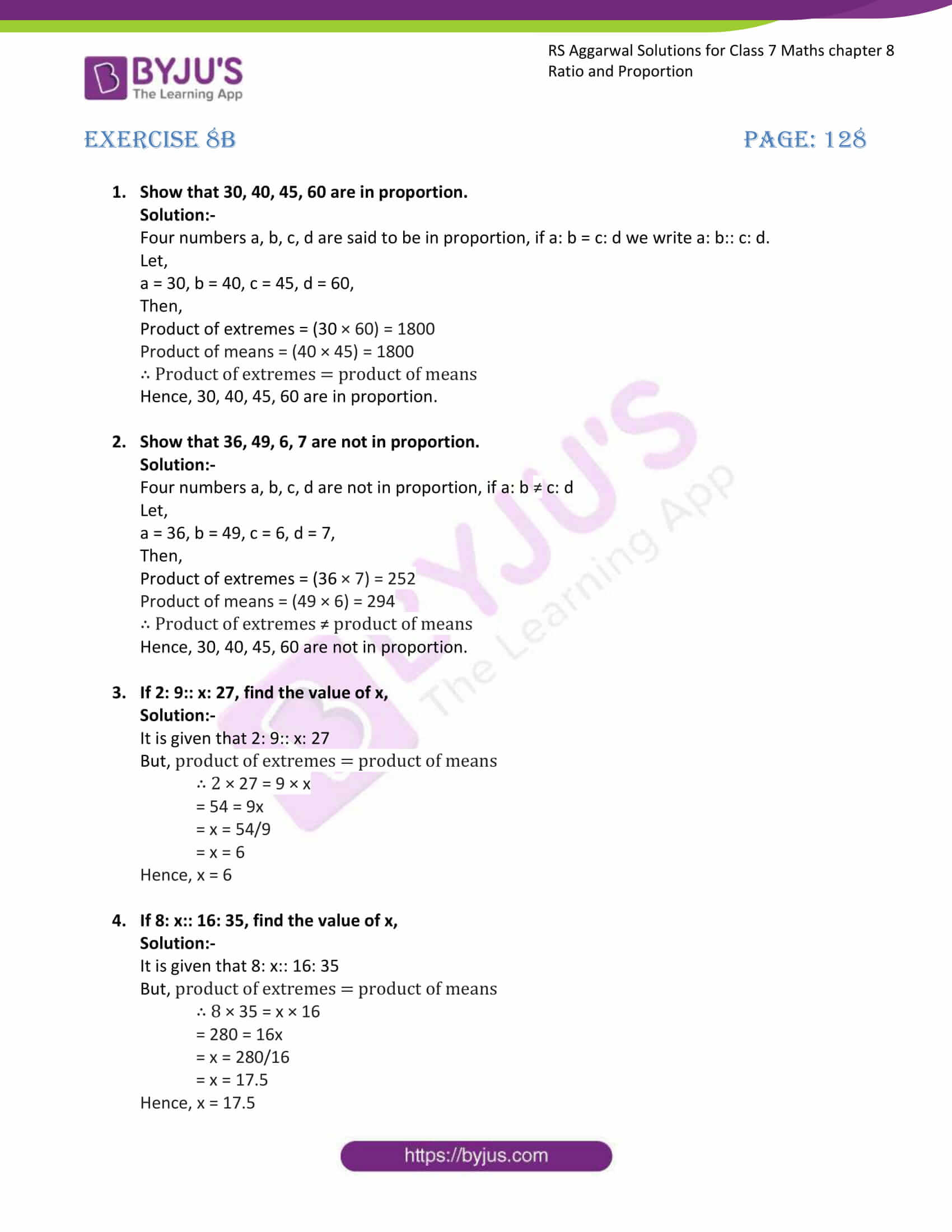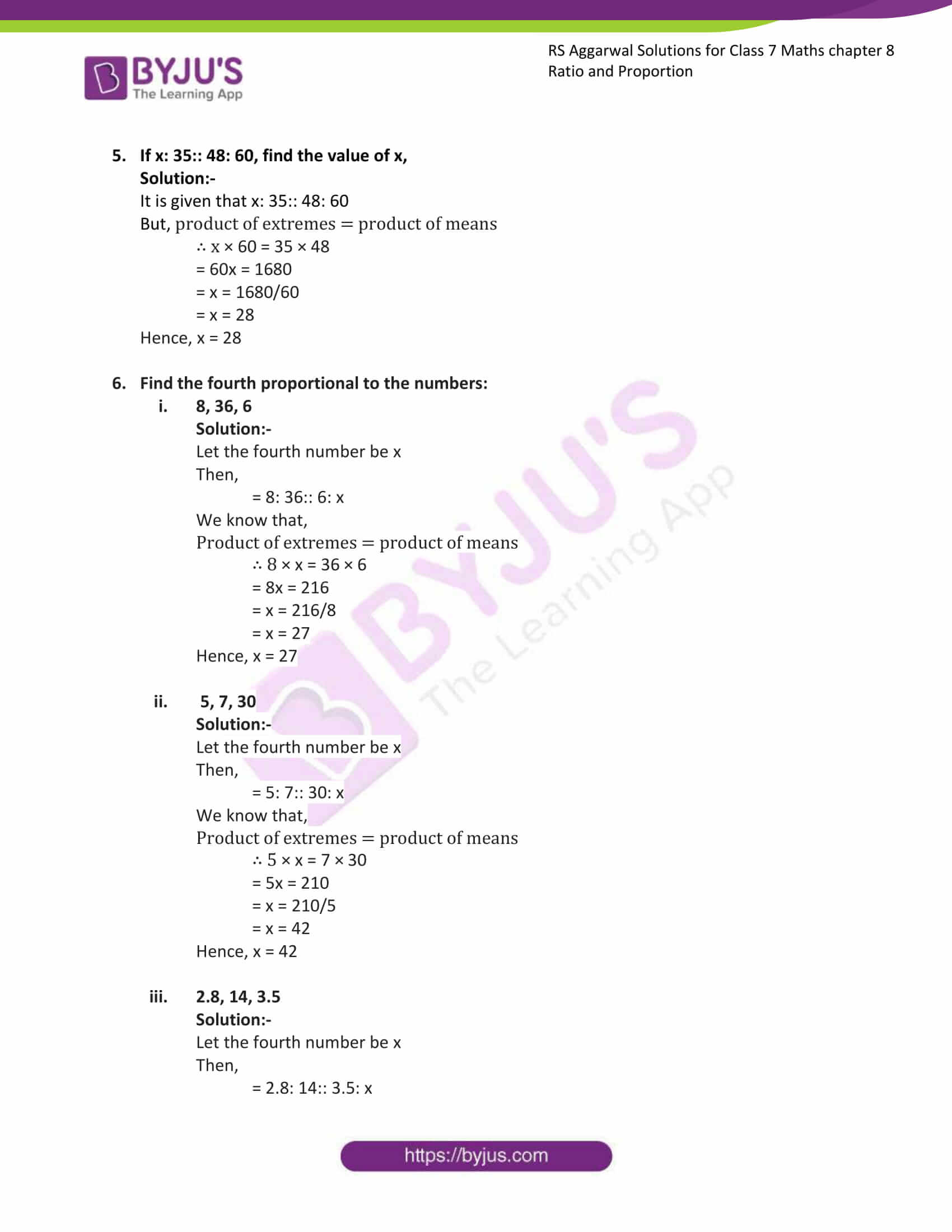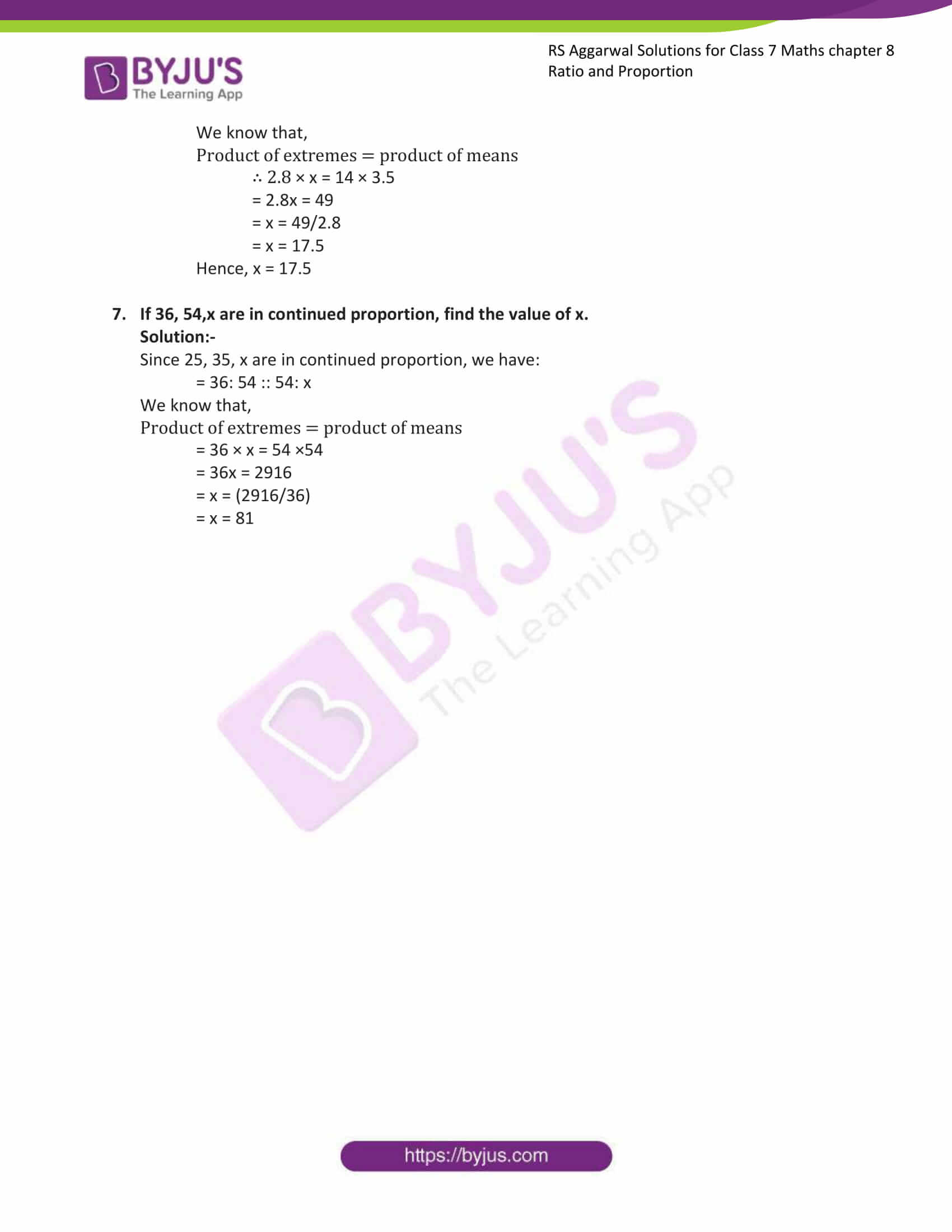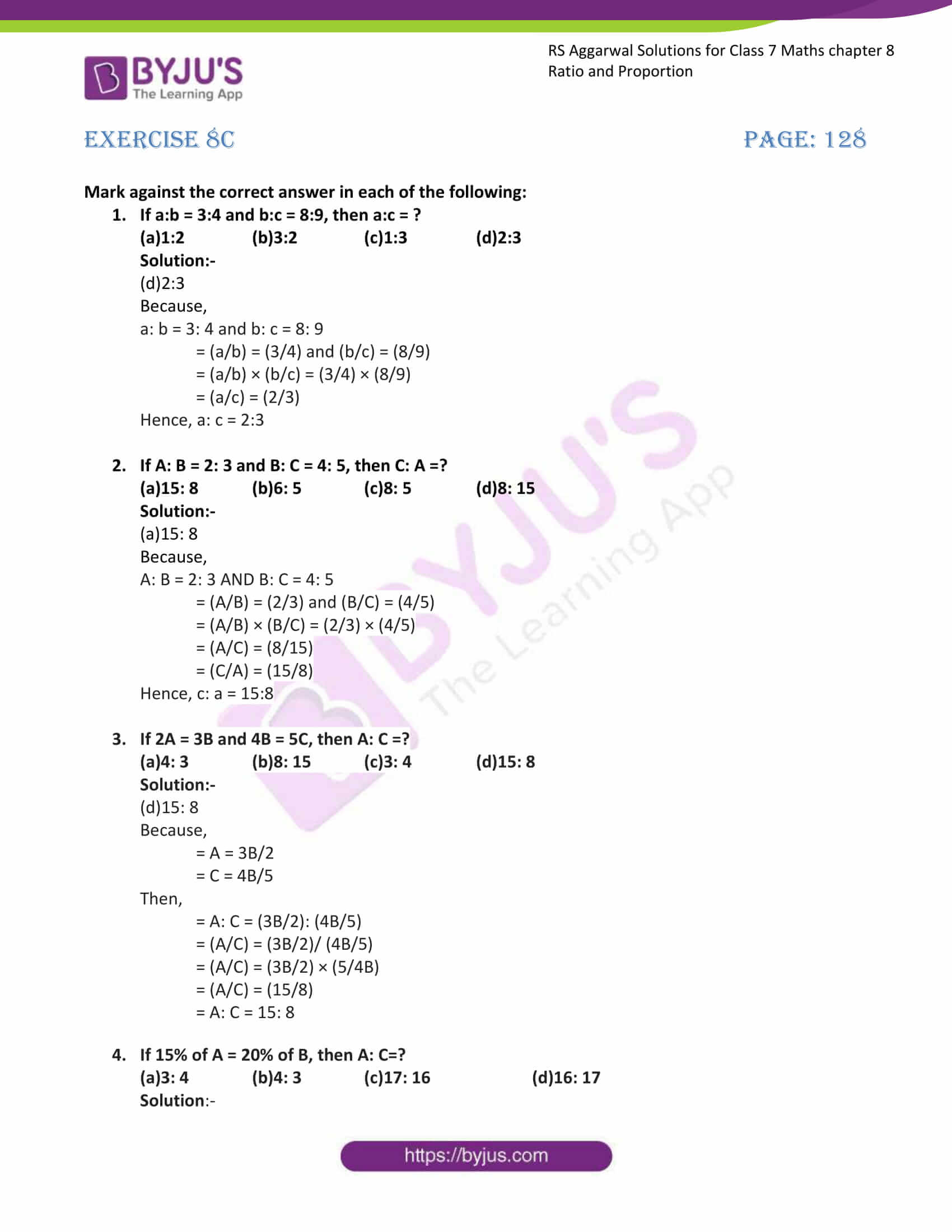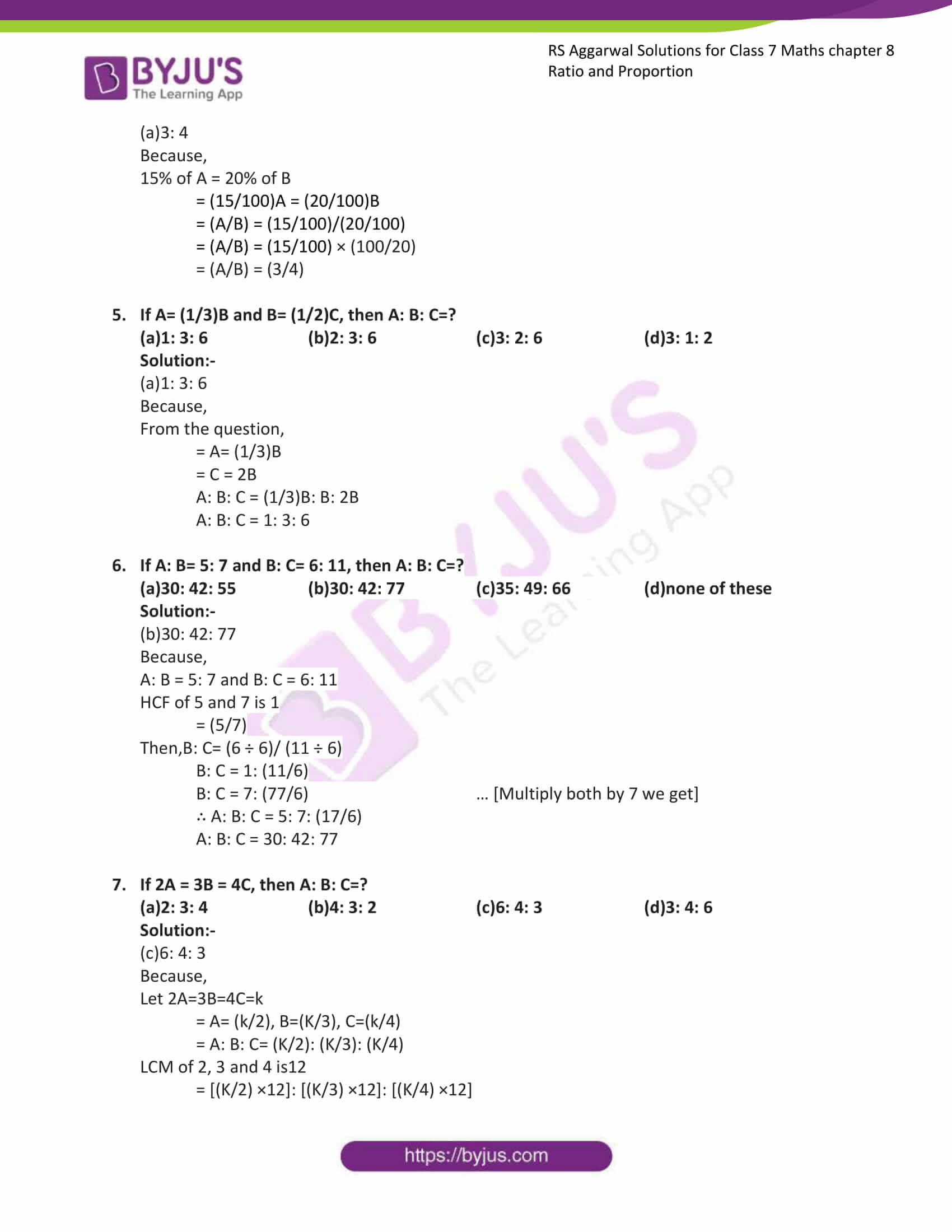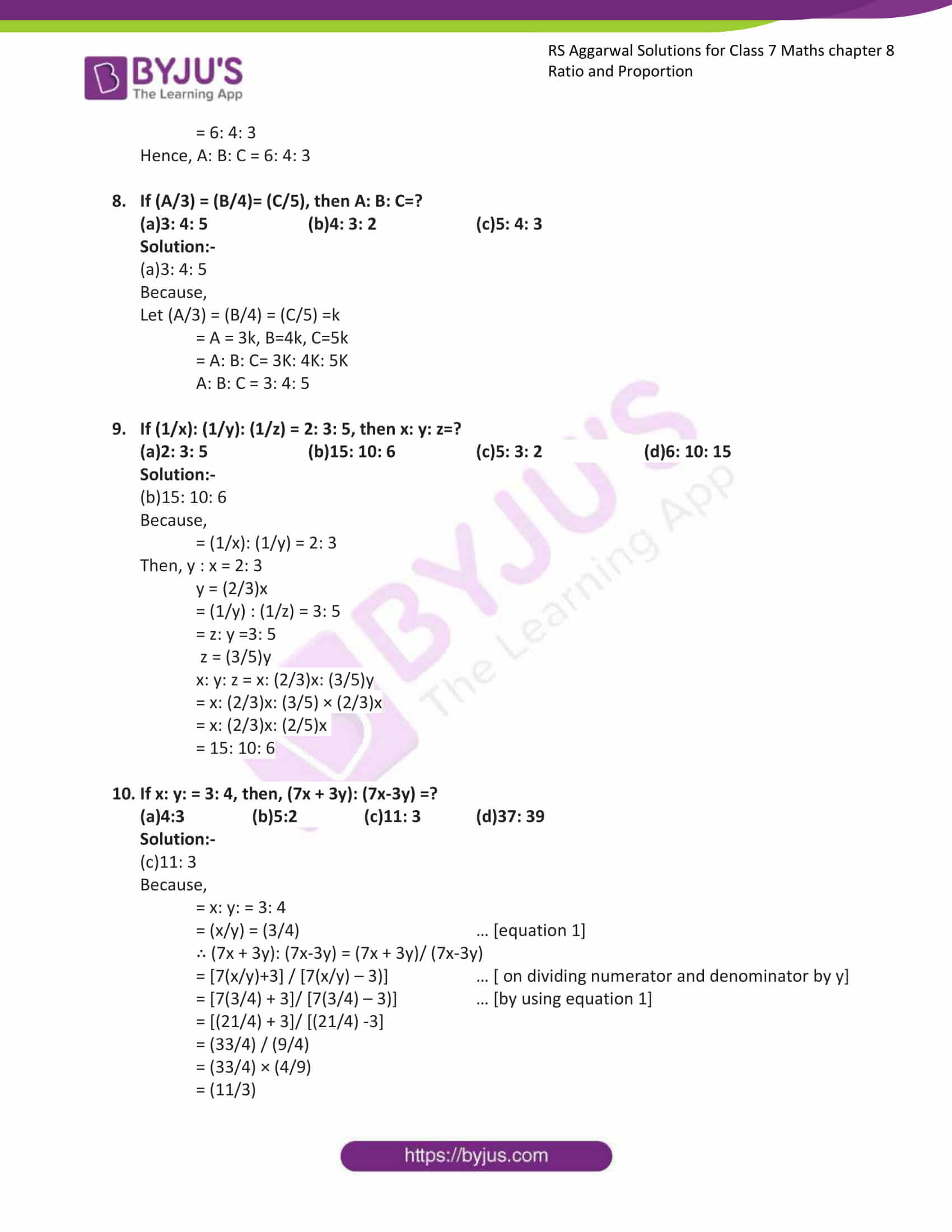Exercise 8A

Exercise 8B

Exercise 8C

### Exercise 8A Page: 124

1. Express each of the following ratios in simplest form:

1. 24 : 40 Solution:-HCF of 24 and 40 is 8

∴ 24: 40 = 24/40

= (24 ÷ 8) / (40 ÷ 4)

= 3/5

= 3:5

Hence the simplest form of 24:40 is 3:5

2. 13.5 : 15Solution:-The given ratio can be written as (13.5/15) = (135/150)

HCF of 135 and 150 is 15

∴ 135: 150 = 135/150

= (135 ÷ 15)/ (150 ÷ 15)

= 9/10

= 9:10

Hence the simplest form of 135:150 is 9:10

3. [6(2/3)] : [7(1/2)]Solution:-First convert the given mixed fraction into improper fraction. [6(2/3)]: [7(1/2)] = (20/3): (15/2)

By cross multiplication, we get,

= 40:45

HCF of 40 and 45 is 5

= (40 ÷ 5) / (45 ÷ 5)

= (8/9)

= 8:9

Hence the simplest form of 40:45 is 8:9

4. (1/6) : (1/9)Solution:-By cross multiplication, we get,

= 9:6

HCF of 9 and 6 is 3

= (9 ÷ 3) / (6 ÷ 3)

= 3/2

= 3:2

Hence the simplest form of 9:6 is 3:2

5. 4 : 5 : (9/2)Solution:-By cross multiplication above question can be written as,

= 4: 5: (9/2) = 8: 10: 9

HCF of 8, 10 and 9 is 1

∴ the simplest form of 8, 10 and 9 is 8:10:9

6. 2.5 : 6.5 : 8Solution:-The given ratio can be written as 2.5: 6.5: 8 = 25: 65: 80

HCF of 25, 65 and 80 is 5

= (25 ÷ 5)/ (65 ÷ 5) / (80 ÷ 5)

= 5/13/16

= 5:13:16

Hence the simplest form of 25:65:80 is 5:13:16

2. Express each of the following ratios in simplest form:

1. 75 paise : 3 rupeesSolution:-Converting both the given quantities in the same units, we have:

We know that,

= 1 rupee = 100paise

∴ 3 rupees = 300 paise

Then,

= 75:300

HCF of 75 and 300 is 75

= (75 ÷ 75) / (300 ÷ 75)

= 1/4

= 1paise: 4paise

2. 1m 5cm : 63cmSolution:-Converting both the given quantities in the same units, we have:

We know that,

= 1m = 100cm

Then,

= (1 × 100) cm + 5cm: 63cm

= 105cm: 63cm

HCF of 105 and 63 is 21

= (105 ÷ 21) / (63 ÷ 21)

= 5/3

= 5cm: 3cm

3. 1 hour 5 minutes : 45 minutesSolution:-Converting both the given quantities in the same units, we have:

We know that,

= 1 hour = 60 minutes

Then,

= (1 × 60) minutes + 5 minutes: 45 minutes

= 65 minutes: 45 minutes

HCF of 65 and 45 is 5

= (65 ÷ 5) / (45 ÷ 5)

= 13/9

= 13minutes: 9minutes

4. 8months : 1yearSolution:-Converting both the given quantities in the same units, we have:

We know that,

= 1 year = 12 months

Then,

= 8 months: (1 × 12) months

= 8: 12

HCF of 8 and 12 is 4

= (8 ÷ 4) / (12 ÷ 4)

= 2/3

= 2months: 3months

5. (2kg 250g) : (3kg)Solution:-Converting both the given quantities in the same units, we have:

We know that,

= 1 kg = 1000g

Then,

= (2 × 1000) g + 250g: (3 × 1000) g

= 2250g: 3000g

HCF of 2250 and 3000 is 750

= (2250 ÷ 750) / (3000 ÷ 750)

= (3/4)

= 3 g: 4g

6. 1km : 750mSolution:-Converting both the given quantities in the same units, we have:

We know that,

= 1 km = 1000m

Then,

= (1 × 1000) m: 750m

= 1000m: 750m

HCF of 1000 and 750 is 250

= (1000 ÷ 250) / (750 ÷ 250)

= (4/3)

= 4m: 3m

3. If A: B = 7: 5 and B: C = 9: 14, find A: C.

Solution:-

A: B = 7: 5 and B: C = 9: 14

= (A/B) = (7/5) and (B/C) = (9/14)

= (A/B) × (B/C) = (7/5) × (9/14)

= (A/C) = (9/10)

Hence, A: C = 9:10

4. If A: B = 5: 8 and B: C = 16: 25, find A: C.

Solution:-

A: B = 5: 8 and B: C = 16: 25

= (A/B) = (5/8) and (B/C) = (16/25)

= (A/B) × (B/C) = (5/8) × (16/25)

= (A/C) = (2/5)

Hence, A: C = 2:5

5. If A: B =3: 5 and B: C = 10: 13, find A: B: C.

Solution:-

A: B = 3: 5 and B: C = 10: 13

HCF of 3 and 5 is 1

= (3/5)

Then,

B: C= (10 ÷ 2)/ (13 ÷ 2)

B: C = 5: (13/2)

∴ A: B: C = 3: 5: (13/2)

A: B: C = 6: 10: 13

6. If A: B =5: 6 and B: C = 4: 7, find A: B: C.

Solution:-

A: B = 5: 6 and B: C = 4: 7

HCF of 5 and 6 is 1

= (5/6)

Then,

B: C= (4 × (6/4))/ (7 × (6/4))

B: C = (6 / (21/2))

B: C = 6: (21/2)

∴ A: B: C = 5: 6: (21/2)

A: B: C = 10: 12: 21

7. Divide ₹360 between kunal and Mohit in the ratio 7: 8.

Solution:-

From the question,

Sum of the ratios 7: 8 = 7 + 8 = 15

Total share kunal has = ₹ 360 × (7/15) = ₹168

Total share Mohit has = ₹ 360 × (8/15) = ₹192

8. Divide ₹880 between Rajan and kamal in the ratio (1/5): (1/6)

Solution:-

From the question,

Sum of the ratios (1/5): (1/6)

= (1/5) + (1/6)

= (6 + 5)/30

= 11/30

Total share Rajan has = ₹880 × [(1/5) / (11/30)]

= 880 × [(1/5) × (30/11)]

= 880 × (6/11)

= ₹480

Total share kamal has = ₹880 × [(1/6) / (11/30)]

= 880 × [(1/6) × (30/11)]

= 880 × (5/11)

= ₹400

9. Divide ₹5600 between A, B and C in the ratio 1: 3: 4

Solution:-

From the question,

Sum of the ratios 1: 3: 4 = 1 + 3 + 4 = 8

Total share A has = ₹ 5600 × (1/8) = ₹700

Total share B has = ₹ 5600 × (3/8) = ₹2100

Total share c has = ₹ 5600 × (4/8) = ₹2800

10. What number must be added to each term of the ratio 9: 16 to make the ratio 2: 3?

Solution:-

Let the number be x,

Then the two term becomes,

= (9 + x): (16 + x) = 2: 3

= (9 + x) / (16 + x) = (2/3)

By cross multiplication,

= 3 × (9 + x) = 2 × (16 + x)

= 27 + 3x = 32 + 2x

Transposing 27 to RHS and 2x to LHS

= 3x – 2x = 32 – 27

= x = 5

Hence, the number be added to each of the ratio 9:16 to make the ratio 2: 3 is 5

11. What number must be subtracted from each term of the ratio 17: 33 so that the ratio becomes 7: 15?

Solution:-

Let the number be x,

Then the two term becomes,

= (17 – x): (33 – x) = 7: 15

= (17 – x) / (33 – x) = (7/15)

By cross multiplication,

= 15 × (17 – x) = 7 × (33 – x)

= 255 – 15x = 231 – 7x

Transposing -15x to RHS and 231 to LHS

= 255 – 231 = 15x -7x

= 8x = 24

= x = (24/8)

= x = 3

Hence, the number be subtracted from each term of the ratio 17:33 to make the ratio 7: 15 is 3

12. Two number are in the ratio 7: 11. If 7 is added to each of the numbers, the ratio becomes 2: 3. Find the numbers.

Solution:-

Let the numbers are 7x and 11x,

Then,

= (7x + 7): (11x + 7) = 2: 3

= (7x + 7)/ (11x + 7) = (2/3)

By cross multiplication,

= 3 × (7x + 7) = 2 × (11x + 7)

= 21x + 21 = 22x + 14

Transposing 21x to RHS and 14 to LHS

= 21 – 14 = 22x – 21

= x = 7

Hence the numbers are 7x = 7 × 7 = 49

11x = 11 × 7 =77

## Exercise 8B

1. Show that 30, 40, 45, 60 are in proportion.

Solution:-

Four numbers a, b, c, d are said to be in proportion, if a: b = c: d we write a: b:: c: d.

Let,

a = 30, b = 40, c = 45, d = 60,

Then,

Product of extremes = (30 × 60) = 1800

Product of means = (40 × 45) = 1800

∴ Product of extremes = product of means

Hence, 30, 40, 45, 60 are in proportion.

2. Show that 36, 49, 6, 7 are not in proportion.

Solution:-

Four numbers a, b, c, d are not in proportion, if a: b ≠ c: d

Let,

a = 36, b = 49, c = 6, d = 7,

Then,

Product of extremes = (36 × 7) = 252

Product of means = (49 × 6) = 294

∴ Product of extremes ≠ product of means

Hence, 30, 40, 45, 60 are not in proportion.

3. If 2: 9:: x: 27, find the value of x,

Solution:-

It is given that 2: 9:: x: 27

But, product of extremes = product of means

∴ 2 × 27 = 9 × x

= 54 = 9x

= x = 54/9

= x = 6

Hence, x = 6

4. If 8: x:: 16: 35, find the value of x,

Solution:-

It is given that 8: x:: 16: 35

But, product of extremes = product of means

∴ 8 × 35 = x × 16

= 280 = 16x

= x = 280/16

= x = 17.5

Hence, x = 17.5

5. If x: 35:: 48: 60, find the value of x,

Solution:-

It is given that x: 35:: 48: 60

But, product of extremes = product of means

∴ x × 60 = 35 × 48

= 60x = 1680

= x = 1680/60

= x = 28

Hence, x = 28

6. Find the fourth proportional to the numbers:

1. 8, 36, 6Solution:-Let the fourth number be x

Then,

= 8: 36:: 6: x

We know that,

Product of extremes = product of means

∴ 8 × x = 36 × 6

= 8x = 216

= x = 216/8

= x = 27

Hence, x = 27

2. 5, 7, 30Solution:-Let the fourth number be x

Then,

= 5: 7:: 30: x

We know that,

Product of extremes = product of means

∴ 5 × x = 7 × 30

= 5x = 210

= x = 210/5

= x = 42

Hence, x = 42

3. 2.8, 14, 3.5Solution:-Let the fourth number be x

Then,

= 2.8: 14:: 3.5: x

We know that,

Product of extremes = product of means

∴ 2.8 × x = 14 × 3.5

= 2.8x = 49

= x = 49/2.8

= x = 17.5

Hence, x = 17.5

4. If 36, 54,x are in continued proportion, find the value of x.Solution:-Since 25, 35, x are in continued proportion, we have:

= 36: 54 :: 54: x

We know that,

Product of extremes = product of means

= 36 × x = 54 ×54

= 36x = 2916

= x = (2916/36)

= x = 81

## Exercise 8C

Mark against the correct answer in each of the following:

1. If a:b = 3:4 and b:c = 8:9, then a:c = ?

(a)1:2 (b)3:2 (c)1:3 (d)2:3

Solution:-

(d)2:3

Because,

a: b = 3: 4 and b: c = 8: 9

= (a/b) = (3/4) and (b/c) = (8/9)

= (a/b) × (b/c) = (3/4) × (8/9)

= (a/c) = (2/3)

Hence, a: c = 2:3

2. If A: B = 2: 3 and B: C = 4: 5, then C: A =?

(a)15: 8 (b)6: 5 (c)8: 5 (d)8: 15

Solution:-

(a)15: 8

Because,

A: B = 2: 3 AND B: C = 4: 5

= (A/B) = (2/3) and (B/C) = (4/5)

= (A/B) × (B/C) = (2/3) × (4/5)

= (A/C) = (8/15)

= (C/A) = (15/8)

Hence, c: a = 15:8

3. If 2A = 3B and 4B = 5C, then A: C =?

(a)4: 3 (b)8: 15 (c)3: 4 (d)15: 8

Solution:-

(d)15: 8

Because,

= A = 3B/2

= C = 4B/5

Then,

= A: C = (3B/2): (4B/5)

= (A/C) = (3B/2)/ (4B/5)

= (A/C) = (3B/2) × (5/4B)

= (A/C) = (15/8)

= A: C = 15: 8

4. If 15% of A = 20% of B, then A: C=?

(a)3: 4 (b)4: 3 (c)17: 16 (d)16: 17

Solution:-

(a)3: 4

Because,

15% of A = 20% of B

= (15/100)A = (20/100)B

= (A/B) = (15/100)/(20/100)

= (A/B) = (15/100) × (100/20)

= (A/B) = (3/4)

5. If A= (1/3)B and B= (1/2)C, then A: B: C=?

(a)1: 3: 6 (b)2: 3: 6 (c)3: 2: 6 (d)3: 1: 2

Solution:-

(a)1: 3: 6

Because,

From the question,

= A= (1/3)B

= C = 2B

A: B: C = (1/3)B: B: 2B

A: B: C = 1: 3: 6

6. If A: B= 5: 7 and B: C= 6: 11, then A: B: C=?

(a)30: 42: 55 (b)30: 42: 77 (c)35: 49: 66 (d)none of these

Solution:-

(b)30: 42: 77

Because,

A: B = 5: 7 and B: C = 6: 11

HCF of 5 and 7 is 1

= (5/7)

Then,B: C= (6 ÷ 6)/ (11 ÷ 6)

B: C = 1: (11/6)

B: C = 7: (77/6) … [Multiply both by 7 we get]

∴ A: B: C = 5: 7: (17/6)

A: B: C = 30: 42: 77

7. If 2A = 3B = 4C, then A: B: C=?

(a)2: 3: 4 (b)4: 3: 2 (c)6: 4: 3 (d)3: 4: 6

Solution:-

(c)6: 4: 3

Because,

Let 2A=3B=4C=k

= A= (k/2), B=(K/3), C=(k/4)

= A: B: C= (K/2): (K/3): (K/4)

LCM of 2, 3 and 4 is12

= [(K/2) ×12]: [(K/3) ×12]: [(K/4) ×12]

= 6: 4: 3

Hence, A: B: C = 6: 4: 3

8. If (A/3) = (B/4)= (C/5), then A: B: C=?

(a)3: 4: 5 (b)4: 3: 2 (c)5: 4: 3

Solution:-

(a)3: 4: 5

Because,

Let (A/3) = (B/4) = (C/5) =k

= A = 3k, B=4k, C=5k

= A: B: C= 3K: 4K: 5K

A: B: C = 3: 4: 5

9. If (1/x): (1/y): (1/z) = 2: 3: 5, then x: y: z=?

(a)2: 3: 5 (b)15: 10: 6 (c)5: 3: 2 (d)6: 10: 15

Solution:-

(b)15: 10: 6

Because,

= (1/x): (1/y) = 2: 3

Then, y : x = 2: 3

y = (2/3)x

= (1/y) : (1/z) = 3: 5

= z: y =3: 5

z = (3/5)y

x: y: z = x: (2/3)x: (3/5)y

= x: (2/3)x: (3/5) × (2/3)x

= x: (2/3)x: (2/5)x

= 15: 10: 6

10. If x: y: = 3: 4, then, (7x + 3y): (7x-3y) =?

(a)4:3 (b)5:2 (c)11: 3 (d)37: 39

Solution:-

(c)11: 3

Because,

= x: y: = 3: 4

= (x/y) = (3/4) … [equation 1]

∴ (7x + 3y): (7x-3y) = (7x + 3y)/ (7x-3y)

= [7(x/y)+3] / [7(x/y) – 3)] … [ on dividing numerator and denominator by y]

= [7(3/4) + 3]/ [7(3/4) – 3)] … [by using equation 1]

= [(21/4) + 3]/ [(21/4) -3]

= (33/4) / (9/4)

= (33/4) × (4/9)

= (11/3)

## RS Aggarwal Solutions for Class 7 Maths Chapter 8 – Ratio and Proportion

Chapter 8 – Ratio and Proportion contain 3 exercises, and the RS Aggarwal Solutions available on this page provide solutions to the questions present in the exercises. Now, let us have a look at some of the concepts explained in this chapter.

• Ratio
• Terms of Ratio
• Comparison of Ratios
• Proportion

### Chapter Brief of RS Aggarwal Solutions for Class 7 Maths Chapter 8 – Ratio and Proportion

RS Aggarwal Solutions for Class 7 Maths Chapter 8 – Ratio and Proportion. The ratio of two quantities of the same kind and in the same units is a fraction that shows many times the one quantity is of the same kind. Proportion is an equality of two ratios. The solutions are solved in such a way that students will understand clearly and they will never get confused.